# 五分钟搞定删除excel单元格空格的问题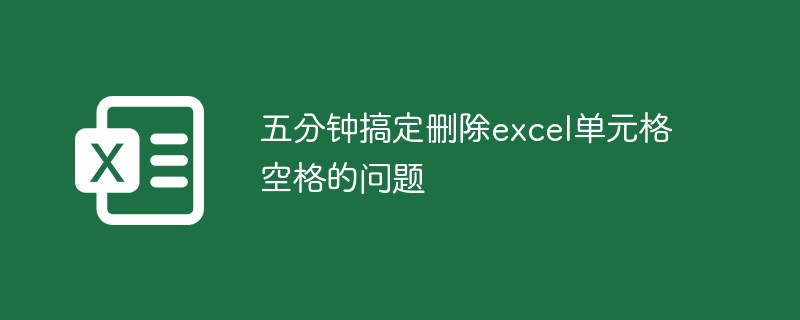## 1、 删除严重空间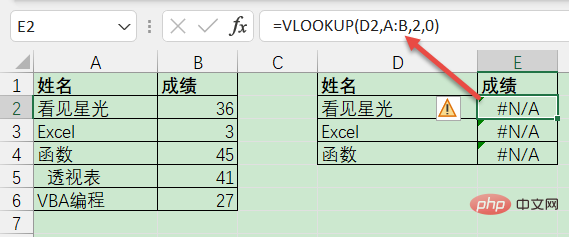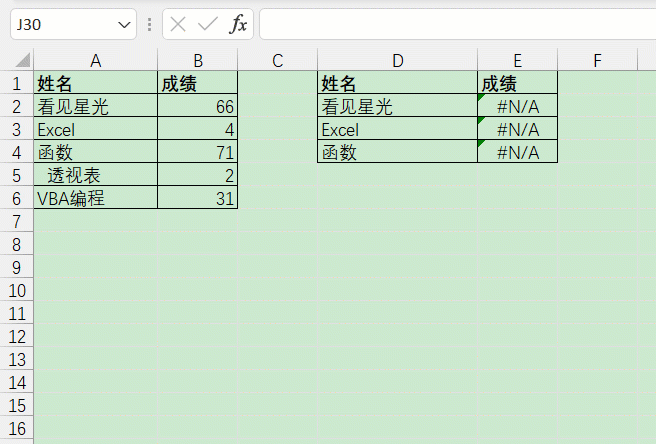## 2、身份证中的空格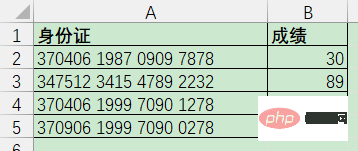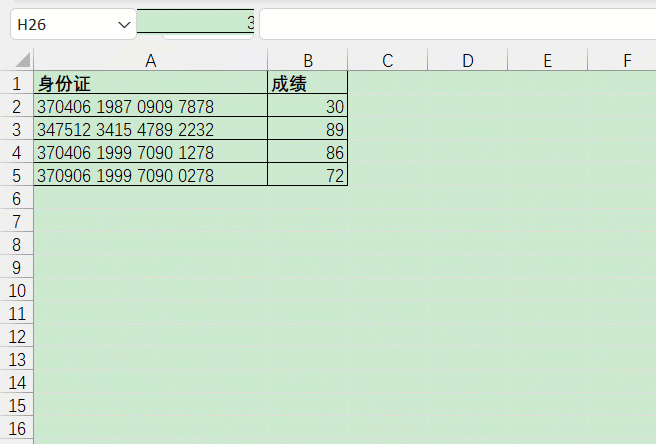`=SUBSTITUTE(A2,`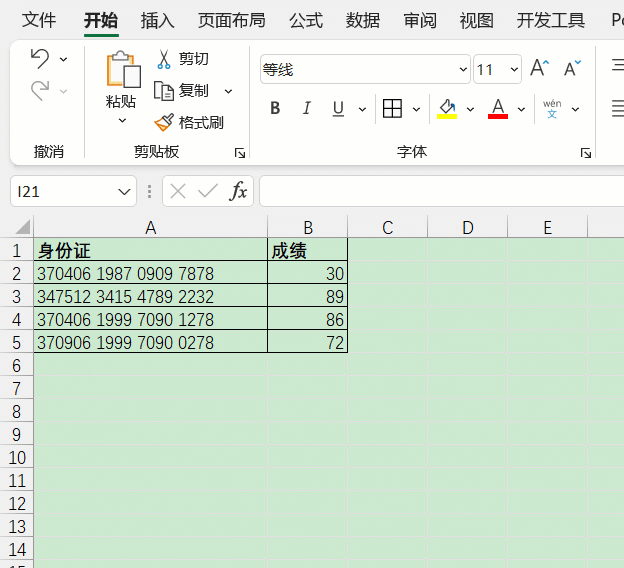## 3、清除首尾空格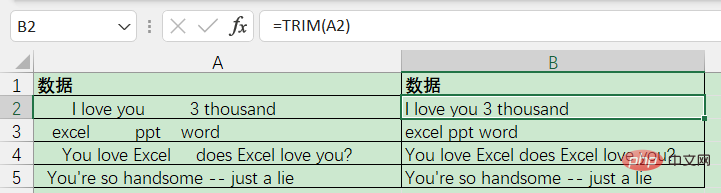B2单元格输入以下公式即可：

`=TRIM(A2)`

## 4、删除系统导出的空间

`=UNICHAR(ROW(A1))`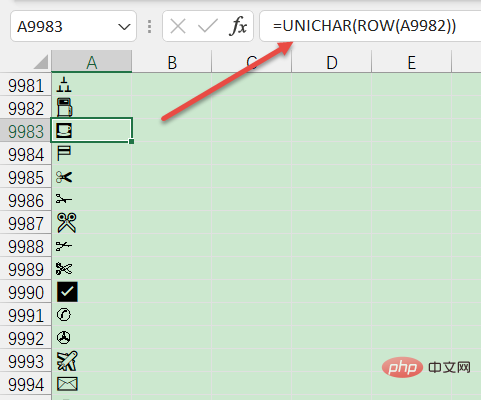`=CLEAN(TRIM(A1))`

CLEAN，在英语中，它意味着清洁，它可以清除一些看不见的空间。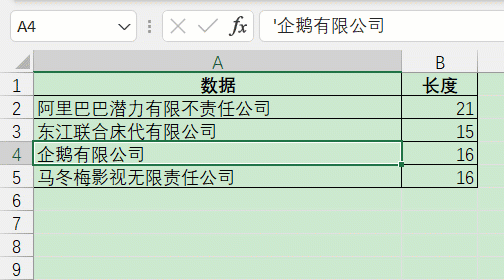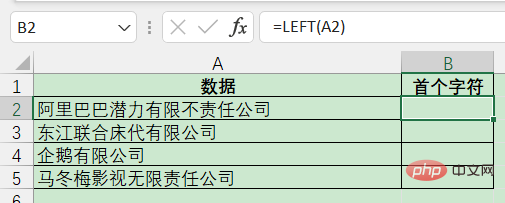`=SUBSTITUTE(A2,LEFT(\$A\$2),””)`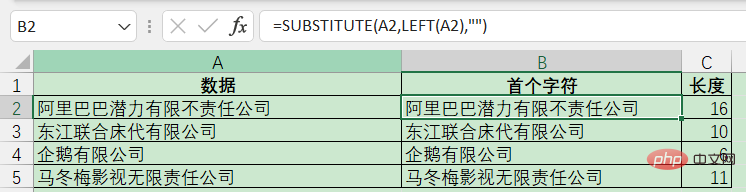`=SUBSTITUTE(A2,RIGHT(\$A\$2),””)`

`=SUBSTITUTE(SUBSTITUTE(A2,RIGHT(\$A\$2),””),LEFT(\$A\$2),””)`